#### Mechanics, Strength of Materials and Theory of Structures

SHEAR FORCE & BENDING MOMENT
PURE BENDING
SOLVED EXAMPLES BASED ON SHEAR FORCE AND BENDING MOMENT DIAGRAMS
FLEXURAL STRESS

## SOLVED EXAMPLES BASED ON SHEAR FORCE AND BENDING MOMENT DIAGRAMS

SOLVED EXAMPLES BASED ON SHEAR FORCE AND BENDING MOMENT DIAGRAMS

19.1 Problem

A cantilever beam is subjected to various loads as shown in figure. Draw the shear force diagram and bending moment diagram for the beam.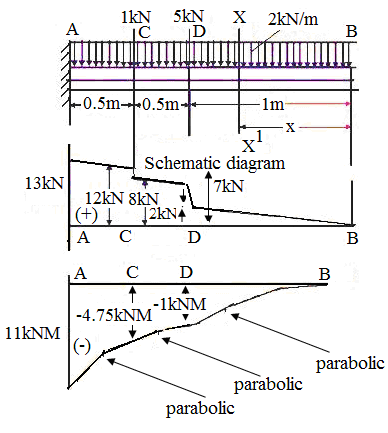Fig. 19.1 Shear force and bending moment

Solution: Consider a section (X – X’) at a distance x from section B. shear force. between B and D;

Shear force Fx = + wx

At x = 0, Fb = 0 …(1)

x = 1 m; Fd just right = 2 × 1 = 2 kN

S.F. between D and C; Fx = + wx + 5

At x = 1 m; FD just left = (2 × 1) + 5 = 7 kN

At x = 1.5 m; Fc just right = (2 × 1.5) + 5 = 8 kN

S.F. between C and A

Fx = + wx + 5 + 4

At x = 1.5 m; Fc just left = 2 × 1.5 + 5 + 4 = 12 kN

At x = 2 m; FA = 2 × 2 + 5 + 4 = 13 kN

Bending moment between C and A;

Mx = – (wx).x/2

Mx = wx2/2 – 5 (x – 1) – 4 (x – 1.5)

At x = 1.5 m; Mc = –2 × (1.5)2 / 2 –5 (1.5 – 1) – 4 (1.5 – 1.5)

Mc = –4.75 kN- m

x = 2.0 m; Ma = – 2 × (2)/ 2 – 5 (2.0 – 1) – 4 (2.0 – 1.5)

Ma = - 11 kN-m

(The sign of bending moment is taken to be negative because the load creates hogging).

The shape of bending moment diagram is parabolic in shape from B to D, D to C, and, also C to A.

19.2 Problem

A cantilever beam carries a uniform distributed load of 60 kN/m as shown in figure. Draw the shear force and bending moment diagrams for the beam.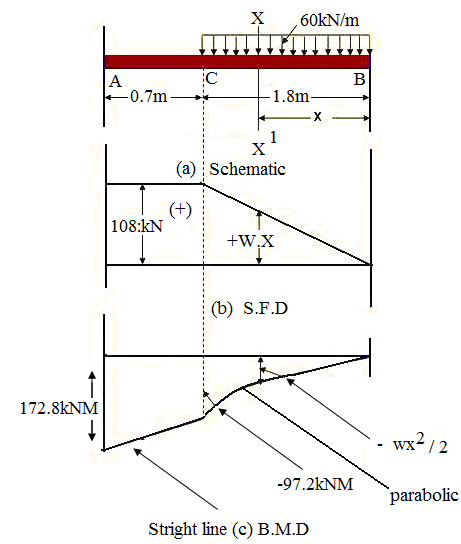Fig. 19.2 Simply supported beam carrying UDL upto some extent

Solution: Consider a section (X – X’) at a distance x from end B.

Shear force = Total unbalanced vertical force on either side of the section.

S.F. between B and C Fx = + w.x …(1)

A x = 0, FB = w.0 = 0

x= 1.8 m; FC = 60 × 1.8 = 108 kN

Since, there is no load between points A and C; for this region Fx remains constant. Therefore, from C to A;

Fx = + 108 kN

(The sign is taken to be positive because the resultant force is in downward direction on right hand side of the section).

SFD will be triangular from B to C and a rectangle from C to A.

Now,

Bending moment between B and C Mx = – (wx).x/2 = –wx2/2

At x = 0; MB = –w.0/2 = 0

x = 1.8 m; MC = –60/2.(1.8)2 = –97.2 kN m

For region C to A; Mx = – w (1.8)(x – 1.8/2) = – 60 × 1.8 (x – 0.9) = –108 (x – 0.9)

At x = 1.8 m; MC = –108 (1.8 – 0.9) = –97.2 kN m

x = 2.5 m; MA = –108 (2.5 – 0.9) = –172.8 kN m

(The sign of bending moment is taken to be negative because the load creates hogging).

BMD is parabolic in nature from B to C and straight line from C to A.

19.3 Problem

A simply supported beam overhanging on one side is subjected to a uniform distributed load of 1 kN/m. Sketch the shear force and bending moment diagrams and find the position of point of contra-flexure.

Solution: Consider a section (X – X’) at a distance x from end C of the beam.

To draw the shear force diagram and bending moment diagram we need RA and RB.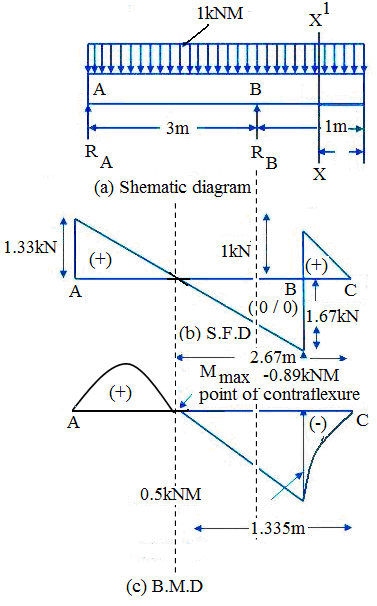Fig. 19.3 simply supported beam carrying -UDL

By taking moment of all the forces about point A.

RB × 3 – w/2 × (4)2 = 0

RB = 1 × (4)2 / 2 × 3 = 8/3 kN

For static equilibrium;

RA + RB – 1 × 4 = 0

RA = 4 – 8/3 = 4/3 kN

To draw shear force diagram we need shear force at all salient points:

Taking a section between C and B, SF at a distance x from end C. we have,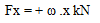At x = 0; FC = 0

x = 1 m; FB just right = 1 × 1 = + 1 kN

Now, taking section between B and A, at a distance x from end C, the SF is: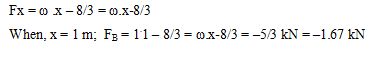At x = 4 m; FA = 4 – 8/3 = + 4/3 kN = + 1.33 kN

The shear force becomes zero;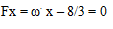═ x = 2.67 m

(The sign is taken positive taken when the resultant force is in downward direction the RHS of the section).

To draw bending moment diagram we need bending moment at all salient points. Taking section between C and B, bending moment at a distance x from end C, we have

Mx = – wx2 / 2 = –1.x2/2 kN m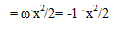When x = 0, MC = 0

At x = 1 m. MB = –1 × (1)2 / 2 = –0.5 kN m

Taking section between B and A, at a distance x from C, the bending moment is:

Mx = –x2/2 + 8/3 (x – 1)

At x = 1 m, MB = – 0.5 kN m

x = 4 m; MA = –(4)2 /2 – 8/3 (4 – 1) = 0

The maximum bending moment occurs at a point where

dMx / dx = 0

═ d/dx [–x2 / 2 + 8/3 x – 8/3] = 0

═ –1/2 × 2x + 8/3 = 0

═ x = 8/3 m from end C.

═ Mmax = –1/2 (8/3)2 + 8/3 (8/3 – 1) = 0.89 kN m

The point of contraflexure occurs at a point, where

Mx = 0

═ –x2/2 + 8/3 (x – 1) = 0

═ x2 = 16/3 (x – 1)

═ x2 – 16/3 x + 16/3 = 0

x = 1.335 m or 4 m

4. A simply supported beam is subjected to a combination of loads as shown in figure. Sketch the shear force and bending moment diagrams and find the position and magnitude of maximum bending moment.

Solution: To draw the shear force diagram and bending moment diagram we need RA and RB.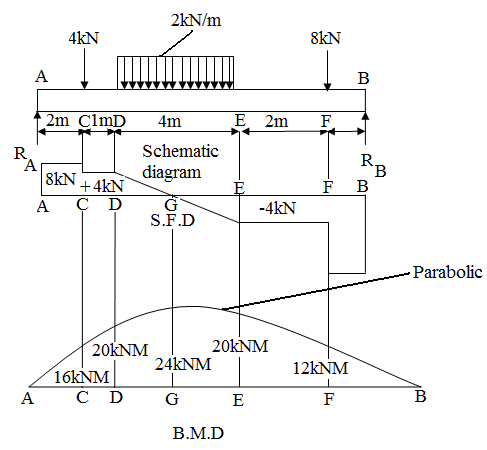Fig. 19.4 Shear force and bending moment

By taking moment of all the forces about point A.

We get RB × 10 – 8 × 9 – 2 × 4 × 5 – 4 × 2 = 0

RB = 12 kN

From condition of static equilibrium ΣFy = 0

RA + RB – 4 – 8 – 8 = 0

RA = 20 – 12 = 8 kN

To draw shear force diagram we need shear force at all salient points:

For AC; FA = + RA = 8 kN

For CD, FC = + 8 – 4 = 4 kN

FD = 4 kN

For DE, Fx = 8 – 4 – 2 (x – 3) = 10 – 2x

At x = 3 m; FD = 10 – 6 = 4 kN

At x = 7 m; FE = 10 – 2 × 7 = –4 kN

The position for zero SF can be obtained by 10 – 2x = 0

x = 5 m

For EF; Fx = 8 – 4 – 8 = – 4 kN

For FB; Fx = 8 – 4 – 8 – 8 = – 12 kN

To draw BMD, we need BM at all salient points.

For region AC, Mx = + 8x

At x = 0; MA = 0

x = 2; MC = 8 × 2 = 16 kN m

For region CD; Mx = + 8x – 4 (x – 2)

At x = 2 m; MC = 8 × 2 – 4 (2 – 2) = 16 kN m

At x = 3 m; MD = 8 × 3 – 4 (3 – 2) = 20 kN m

For region DE,

Mx = + 8x – 4 (x – 2) – 2(x – 3)2 / 2 = 10x – x2 – 1

At x = 3 m; MD = 8 × 3 – 4 (3 – 2) – 2(32 – 3)2 / 2 = 20 kN m

At x = 7 m; ME = 10 × 7 – (7)2 – 1 = 20 kN m

At x = 5 m; MG = 10 × 5 – (5)2 – 1 = 24 kN m

For region EF,

Mx = 8x – 4 (x – 2) – 2 × 4 (x – 5) = 48 – 4x

At x = 9 m, MF = 120 – 12 × 9 = 12 KN m

At x = 10 m; MB = 120 – 12 × 10 = 0

The shear force diagram and bending moment diagram can now be drawn by using the various values of shear force and bending moment. For bending moment diagram the bending moment is proportional to x, so it depends, linearly on x and the lines drawn are straight lines.

The maximum bending moment exists at the point where the shear force is zero, and also dM/dx = 0 in the region of DE

d/dx (10 x – x2 – 1) = 0

10 – 2x = 0

X = 5 m

Mmax = 10 × 5 – (5)2 – 1 = 24 kN m

Thus, the maximum bending is 24 kN m at a distance of 5 m from end A.